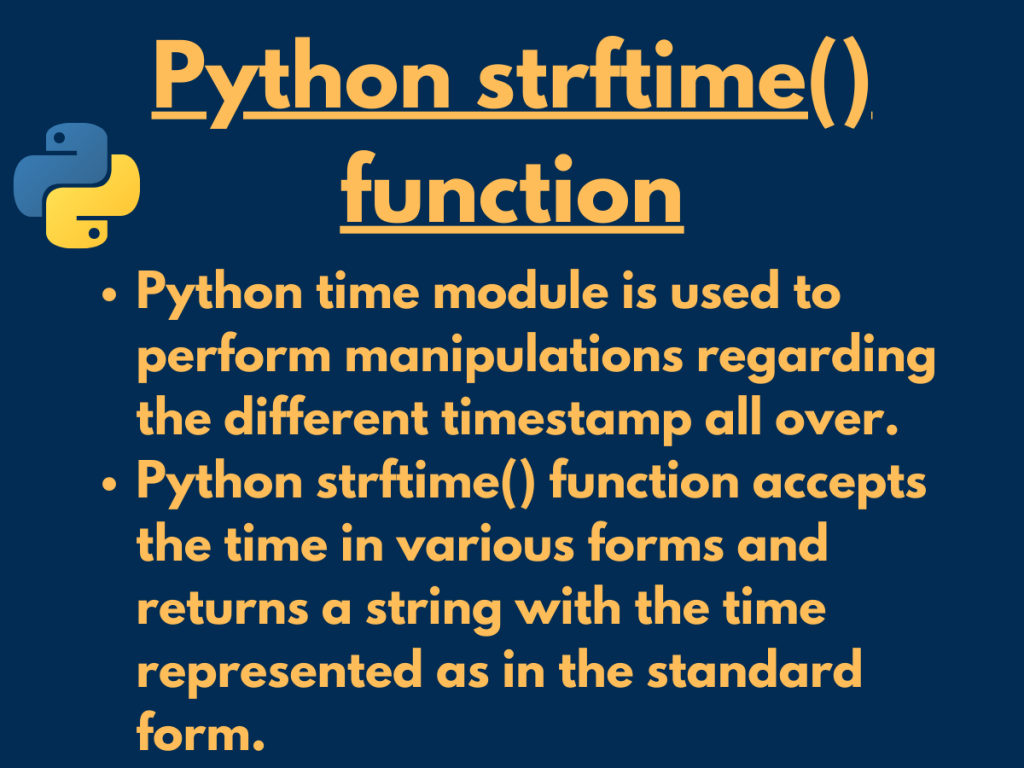# What is Python strftime() function?Hey, folks! In this article, we will be understanding the working of Python strftime() function along with its variations.

So, let us get started.

Python has a variety of modules that have clusters of functions to implement various functionality on the data. Python time module is used to perform manipulations regarding the different timestamp all over.

Further, `Python strftime() function` accepts the time in various forms and returns a string with the time represented as in the standard form.

## Variant 1: Python strftime() to get the current time

Python strftime() function can be used along with the datetime module to fetch the current timestamp in a proper form according to the format codes.

Syntax:

```datetime.now().strftime('format codes')
```

So, format codes are actually the predefined codes used to represent the timestamp in a proper and standard manner. We’ll be understanding more about format codes further in this article.

Example:

```from datetime import datetime

current_timestamp = datetime.now()
tym = current_timestamp.strftime("%H:%M:%S")
date = current_timestamp.strftime("%d-%m-%Y")
print("Current Time:",tym)
print("Current Date:",date)
```

In the above example, we have used `datetime.now() method` to fetch the current timestamp and then have passed the same to the strftime() function to represent the timestamp in a standard format.

We have used format codes carrying the significance as below:

• `%H` – Representing ‘hours‘ in a 24-hour format.
• `%M` – Representing ‘minutes‘ as a decimal number.
• `%S` – Represents the ‘seconds‘ part of the timestamp.

Output:

```Current Time: 16:28:40
Current Date: 28-04-2020
```

## Variant 2: Python strftime() with pre-defined timestamp

It so happens at times, that we need to display the timestamp of the historical datasets. The same can be performed using Python strftime() function.

The `datetime.fromtimestamp()` method is used to fetch the predefined timestamp. Further, strftime() function can be used to represent it in a standard form using various format codes, as explained above.

Syntax:

```datetime.fromtimestamp(timestamp).strftime()
```

Example:

```from datetime import datetime

given_timestamp = 124579923
timestamp = datetime.fromtimestamp(given_timestamp)
tym = timestamp.strftime("%H:%M:%S")
date = timestamp.strftime("%d-%m-%Y")
print("Time according to the given timestamp:",tym)
print("Date according to the given timestamp:",date)
```

Output:

```Time according to the given timestamp: 03:02:03
Date according to the given timestamp: 13-12-1973
```

## Using different Format codes with Python strftime() function

Python strftime() function uses format codes to represent the timestamp in a standard and maintained format. Further, we can use format codes to segregate days, hours, weeks, etc from the timestamp and display the same.

Let’s understand the format codes with the help of some examples.

Example 1: Format code — ‘%A‘ to display the current date of the localtime.

```from time import strftime

day = strftime("%A")
print("Current day:", day)
```

Output:

```Current day: Tuesday
```

Example 2: Format code — ‘%c’ to display the current localtime.

Format code – ‘%c’ is used to display the current localtime which follows the following format:

Day Month Date hours:mins:sec Year

```from time import strftime

day = strftime("%c")
print("Current timestamp:", day)
```

Output:

```Current timestamp: Tue Apr 28 16:42:22 2020
```

Example 3: Format code — ‘%R‘ to represent the time in a 24 hour format.

```from time import strftime

day = strftime("%R")
print("Current time in a 24-hour format:", day)
```

Output:

```Current time in a 24-hour format: 16:44
```

Example 4: Format code — ‘%r’ to display the time in H:M:S format along with the description i.e. AM or PM.

```from time import strftime

day = strftime("%r")
print("Current time -- hours:mins:seconds", day)
```

Output:

```Current time -- hours:mins:seconds 05:05:19 PM
```

Example 5:

```from time import strftime

day = strftime("%x -- %X %p")
print("Local date and time:", day)
```

In the above example, we have used format code ‘%x’ to represent the local timestamp in terms of date and ‘%X’ to represent the local time in the form of H:M:S. The ‘%p’ format code is used to represent whether the timestamp belongs to AM or PM.

Output:

```Local date and time: 04/28/20 -- 17:08:42 PM
```

## Conclusion

Thus, in this article, we have understood the working of Python strftime() function along with the format codes used.

In order to understand the list of available format codes, please find the link to visit the official documentation in the References.